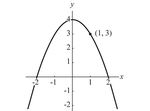# the greatness of logarithms!Which brings us back to logarithms. We need them because it’s always useful to have tools that can undo one another. Just as every office worker needs both a stapler and a staple remover, every mathematician needs exponential functions and logarithms. They’re “inverses.” This means that if you type a number x into your calculator, and then punch the 10x button followed by the log x button, you’ll get back to the number you started with. Logarithms are compressors. They’re ideal for taking numbers that vary over a wide range and squeezing them together so they become more manageable. For instance, 100 and 100 million differ a million-fold, a gulf that most of us find incomprehensible. But their logarithms differ only fourfold (they are 2 and 8, because 100 = 102 and 100 million = 108). In conversation, we all use a crude version of logarithmic shorthand when we refer to any salary between \$100,000 and \$999,999 as being “six figures.” That “six” is roughly the logarithm of these salaries, which in fact span the range from 5 to 6. As impressive as all these functions may be, a mathematician’s toolbox can only do so much — which is why I still haven’t assembled my Ikea bookcases.

more from Steven Strogatz at The Opinionater here.